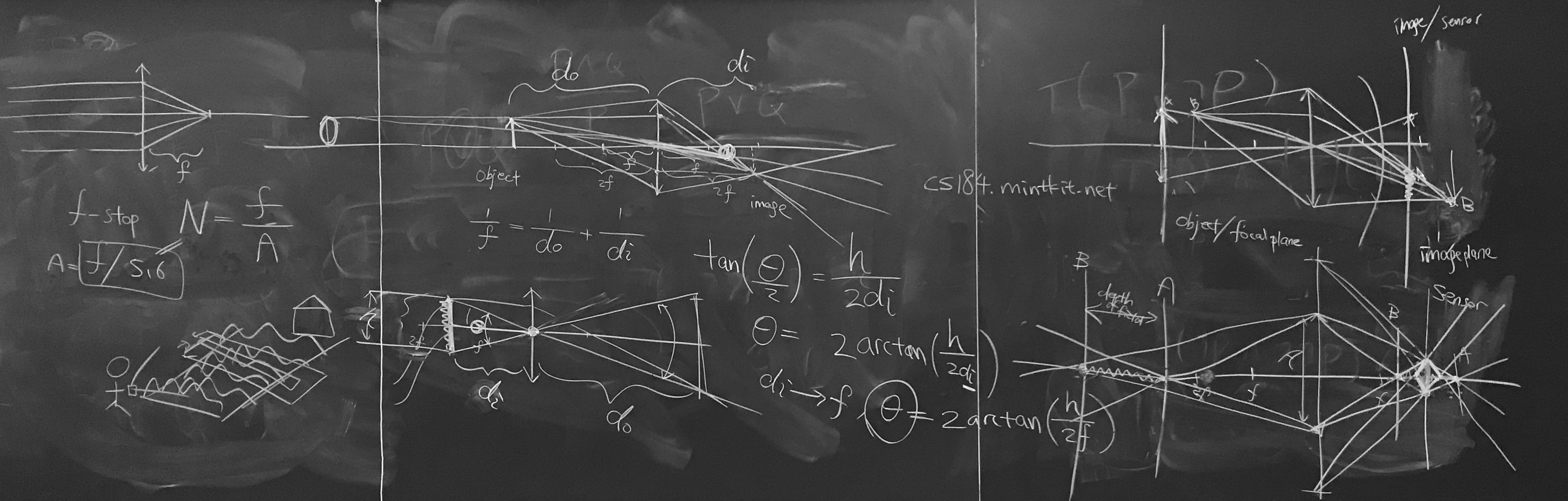Discussion 9

Bullets

• Terminology
• Focal length $f$
• Field of view $fov$
• Exposure
• Shutter speed
• Aperture $A$
• F-stop $N = \frac{f}{A}$
• Circle of confusion
• Depth of field
• Field of view
• Dolly zoom
• Relate field of view to focal length $fov = 2 \arctan(\frac{h}{2f})$
• Exposure
• Time * irradiance
• F stop & shutter stop
• Thin lens approximation
• Gaussian thin lens formula
$\frac{1}{f} = \frac{1}{d_o} + \frac{1}{f_i}$
• Depth of field
• Circle of confusion
• Light field
• Digital refocusing
• Resolution
• Aperture plane resolution dependent on the number of pixels under each microlens
• Microlens plane resolution dependent on the number of microlens
• Sub-aperture image
Represents an image taken with a pinhole camera at the location on the aperture plane

🖍 Board work for everythingBoard work at the end of section